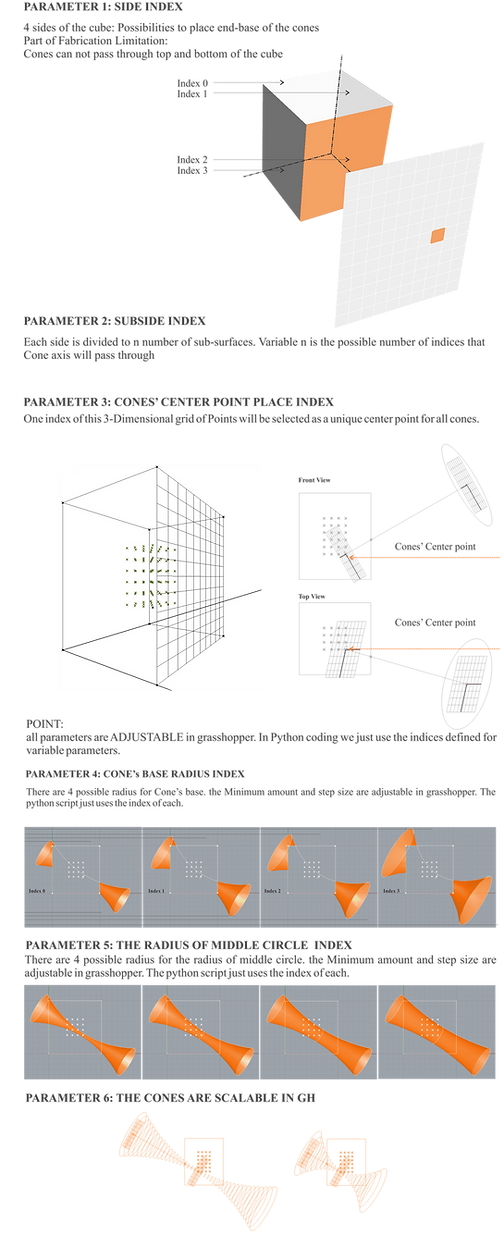## Carnegie Mellon University- Digital Fabrication Lab## INITIAL SET OF INDIVIDUALS## in step 2, for having the second generation, runing the python file in Komodo named “modularity.py”This set is saved in following address and will be read by grasshopper when we run that."c:\\module.txt"Back to step 2 and do all mentioned steps you have done first for each generation

import random
import pickle
from math import *

def checkLimitations(indiv):

#(none of cones should pass through top and bottom sides of cube)
ss=indiv
if ss/24==0:
mini=3
maxi=6
elif ss/24==1:
mini=3
maxi=6
elif ss/24==2:
mini=4
maxi=8
elif ss/24==3:
mini=5
maxi=9
elif ss/24==4:
mini=7
maxi=10
elif ss/24==5:
mini=7
maxi=10
for i in range(n):
if ((indiv[i*4+3] / 10)>=mini and (indiv[i*4+3]/10<=maxi)):
return False
return True

# generates two new children from two selected parents
def crossover(a1,a2,n):
c1=random.randint(0,4*n-1)

# generates the first pivot point (each cone has 4 variables)
c2=random.randint(c1,4*n) # generates second pivot point
ax1=[]
ax2=[]
for i in range(len(a1)):
# selects outside of domain [c1,c2] for first child and inside of domain for the second child (first parent)
# selects inside of domain [c1,c2] for first child and outside of domain for the second child (second parent)
if (i>c1 and i<c2):
ax1+=[a2[i]]
ax2+=[a1[i]]
else:
ax1+=[a1[i]]
ax2+=[a2[i]]
return (ax1,ax2)

# manipulates one of individuals gene
def mutation(a,n):
index=random.randint(0,n-1)*4+random.randint(0,3)+1 # random.randint(0,n-1) selects the cone number, random.randint(0,3) selects which variable of cone should be changed
a[index]=random.randint(0,4)
return a

# selects the top most optimum quarter of individuals
def selection(a,mod):
b=[]    #top quarter (1/4) individuals with highest modularity
modx=sorted(mod)
for i in range(len(a)):
if mod[i]>=modx[len(a)-len(a)/4-1] and mod[i] is not None:
b+=[a[i]]
return (b,max(mod),a[mod.index(max(mod))]) #top quarter individuals, maximum modularity, individual corresponding to the maximum modularity

cp = 96 # number of center points which cones are merged
mod=[]
pkl_file = open("c:\\cone_num.txt", 'rb')
pkl_file.close()

pkl_file = open("c:\\num_indiv.txt", 'rb') #number of individuals in each step
pkl_file.close()

pkl_file = open("c:\\indiv.txt", 'rb')
pkl_file.close()

pkl_file = open("c:\\module.txt", 'rb')
for i in range(len(b)):
pkl_file.close()
#selects the top most quarter of last generation

(b,maxmod,opt_indiv)=selection(b,mod)
# generates the 3/4 of remaining next generation from previous generation

for i in range(3*num_tests/8):
i1=random.randint(0,num_tests/4-1) # index of first parent
i2=random.randint(0,num_tests/4-1) # index of second parent
(cr1,cr2)=crossover(b[i1],b[i2],n) # generates two new individuals from selected parents
# each mutation is for one of new generated individuals

temp = mutation(cr1,n)
if checkLimitations(temp): # checks if new generated individual meets the limitations (none of cones should pass through top and bottom sides of cube)
b+=[temp]  #adding next generation after crossover and mutation
temp = mutation(cr2,n)
if checkLimitations(temp): # checks if new generated individual meets the limitations (none of cones should pass through top and bottom sides of cube)
b+=[temp]  #adding next generation after crossover and mutation

# new generation of individuals are saving in the following file
output = open("c:\\indiv.txt", "wb")
pickle.dump(b, output)
output.close()
print b

output2 = open("c:\\module.txt", "wb") # clears previous data in the file
output2.close()#!/usr/bin/env python